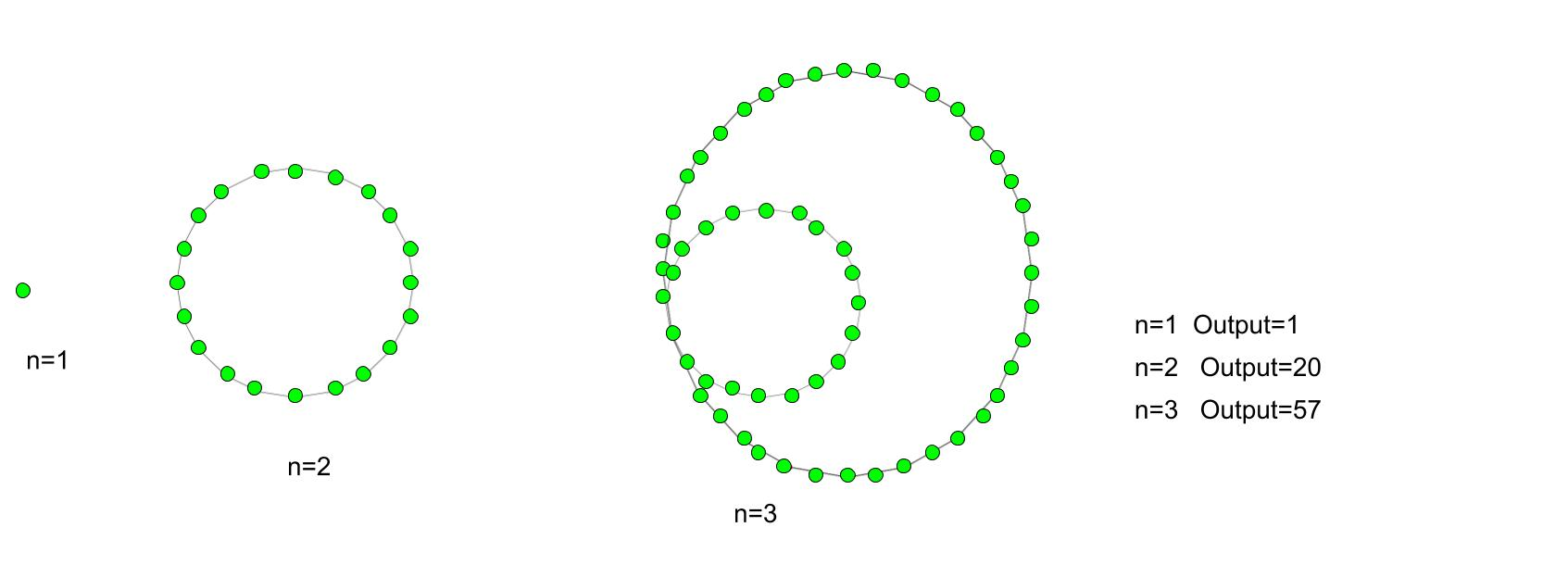Related Articles

# Icosagonal number

• Last Updated : 16 Jul, 2021

Given a number n, the task is to find the nth Icosagonal number.
An Icosagonal number is the 20-gon is a twenty-sided polygon. The number derived from the figurative class. There are different pattern series number in this number. The dots are countable, arrange in a specific way of position and create a diagram. All the dots have a common dots points, all others dots are connected to this points and except this common point the dots connected to their i-th dots with their respective successive layer.
Examples :

Input : 3
Output :57
Input :8
Output :512Formula for nth icosagonal number:## C++

 // C++ program to find// nth Icosagonal number#include using namespace std; // Function to calculate Icosagonal numberint icosagonal_poly(long int n){    // Formula for finding    // nth Icosagonal number    return (18 * n * n - 16 * n) / 2;} // Drivers codeint main(){    long int n = 7;    cout << n << "th Icosagonal number :"               << icosagonal_poly(n);     return 0;}

## Java

 // Java program to find// nth Icosagonal number import java.io.*; class GFG {     // Function to calculate Icosagonal number static int icosagonal_poly(int n){    // Formula for finding    // nth Icosagonal number    return (18 * n * n - 16 * n) / 2;} // Drivers code         public static void main (String[] args) {        int n = 7;         System.out.print (n + "th Icosagonal number :");    System.out.println(icosagonal_poly(n));    }}// This code is contributed by aj_36

## Python 3

 # Python 3 program to find# nth Icosagonal number # Function to calculate# Icosagonal numberdef icosagonal_poly(n) :         # Formula for finding    # nth Icosagonal number    return (18 * n * n -            16 * n) // 2 # Driver Codeif __name__ == '__main__' :    n = 7    print(n,"th Icosagonal number : ",                   icosagonal_poly(n)) # This code is contributed m_kit

## C#

 // C# program to find// nth Icosagonal numberusing System; class GFG{ // Function to calculate// Icosagonal numberstatic int icosagonal_poly(int n){    // Formula for finding    // nth Icosagonal number    return (18 * n * n -            16 * n) / 2;} // Driver codestatic public void Main (){     int n = 7; Console.Write(n + "th Icosagonal " +                        "number :");Console.WriteLine(icosagonal_poly(n));}} // This code is contributed by ajit

## PHP

 

## Javascript

 

Output :

7th Icosagonal number :385

Time Complexity: O(1)
Auxiliary Space: O(1)

Attention reader! Don’t stop learning now. Get hold of all the important DSA concepts with the DSA Self Paced Course at a student-friendly price and become industry ready.  To complete your preparation from learning a language to DS Algo and many more,  please refer Complete Interview Preparation Course.

In case you wish to attend live classes with experts, please refer DSA Live Classes for Working Professionals and Competitive Programming Live for Students.

My Personal Notes arrow_drop_up